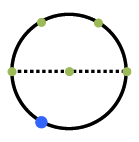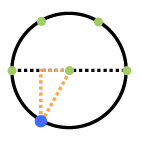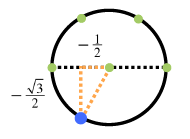### Home > PC > Chapter 4 > Lesson 4.1.2 > Problem4-24

4-24.

Find the following values using special right triangles (give exact values). Do not use your unit circle, except to check.

1. $\text{sin }\frac { 4 \pi } { 3 }$

1. $\text{cos }\frac { 11 \pi } { 3 }$

1. $\text{sin }( - \frac { 5 \pi } { 6 } )$

1. $\text{cos }( - \frac { 2 \pi } { 3 } )$1. Draw circle and locate the angle. Circle with dashed, horizontal diameter, 5 green points located as follows: on left & right ends of diameter, at center of circle, & 2 points equally spaced, on top half. Blue point on the lower left quarter of the circle.

2. Draw a right triangle whose base is the $x$-axis.  Added: right triangle in fourth quadrant: horizontal leg, on the negative, x axis, hypotenuse, from center of circle to blue point.

3. Label side lengths. Labels added: horizontal leg, negative 1 half, vertical leg, negative 1 half times square root of 3.

4. Choose the $y$-value for the sine!